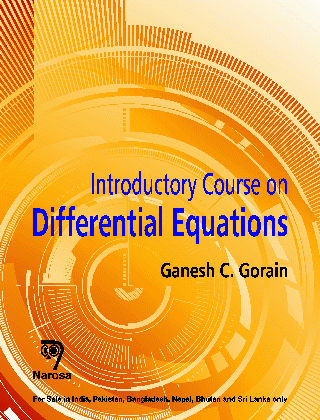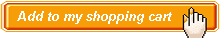Introductory Course on Differential Equations Authors:   Ganesh C. Gorain ISBN: 978-81-8487-399-3 Publication Year:   2014 Pages:   598 Binding:   Paper Back About the book Introductory Course on DIFFERENTIAL EQUATIONS provides an excellent exposition of the fundamentals of ordinary and partial differential equations and is ideally suited for a first course of undergraduate students of mathematics, physics and engineering. The aim of this book is to present the elementary theories of differential equations in the forms suitable for use of those students whose main interest in the subject are based on simple mathematical ideas. Key Features • Discusses the subject in a systematic manner without sacrificing mathematical rigour. • A variety of exercises drill the students in problem solving in view of the mathematical theories explained in the book. • Worked out examples illustrated according to the theories developed in the book with possible alternatives. • Exhaustive collection of problems and the simplicity of presentation differentiates this book from several others. • Material contained will help teachers as well as aspiring students of different competitive examinations. Table of content Introduction to Ordinary Differential Equations / Equations of First Order and First Degree / Equations of First Order and Higher Degree / Applications of First Order Equations / Second Order Linear Equations / Linear Equation of Order n / Simultaneous Linear Equations / Total or Pfaffian Differential Equations / Introduction to Partial Differential Equations / First Order Partial Differential Equations / Laplace Transforms / Bibliography / Index.Audience Undergraduate and Postgraduate Students and Researchers